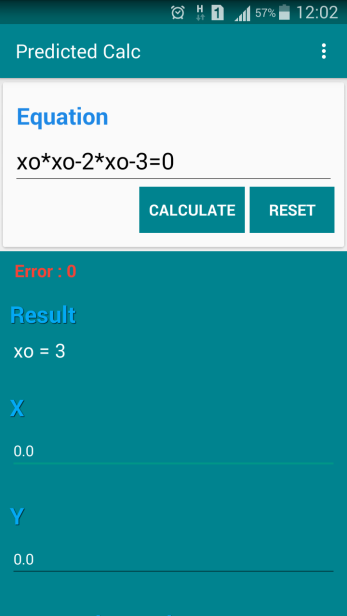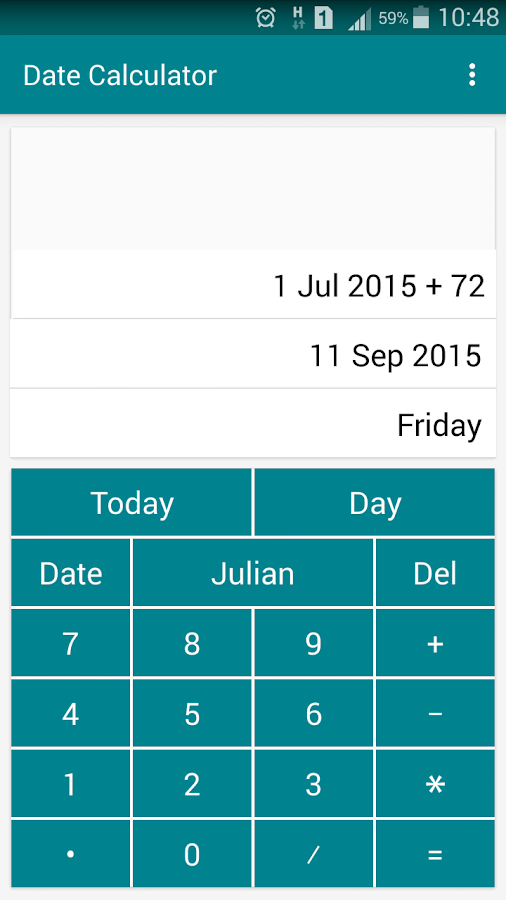## Posts

Showing posts from July, 2015

### The Easy Way to Calculate Root of Polynomial Equation with Predicted CalcPredicted Calc is a calculator that made by android base, and use genetic algorithm to get unknown value of variables that typed on the equation.  Therefore, this app can also be use for finding root of polynomial equation, like an examples below: For example to find root of equation: x2-2*x-3=0 The steps is: Copy and write the equation without x and y, and with math sign operation, for example by using xo, so the equation can be writed as xo*xo-2*xo-3=0, because  x and y was used by Predicted Calc as input variables. Press calculate botton to predict one of roots. Wait until Predicted Calc, approach the best value can be reach. Because using prediction method, Predicted Calc give error result, this error show how far the prediction give best value that can be reach by your device on that calculation, another calculation could be give the different result as same as or more better error value. Predicted Calc will give the result -1 and 3 with error=0, like screenshot below: For prediction ever…

### Date CalculatorOne more, Computational Lab., Dept. of Physics, UB release new android app today, 1 Juli 2015. This app called as Date Calculator and have functionality as general calculator, but with different method where the calculation is based on date data. It's that mean all math operation on this app is basically to optimize user to do math calculation accordingly date math operation. And so in this calculator we will not find minus value, because in Julian Date the minimum value is zero.

Figure 1.
The main idea of Date Calculator is because mostly of us do math calculation based on date data. especially for give dateline to some project, with just type number of day that given, we need to make sure what the date is it happen. However the math operation is the basic operation likeaddition, subtraction, multiplicationanddivision, but if we directly calculate manually, sometimes could be little difficult and wehave to see calender to validate.

For example, like in screenshot Figure 1, to lo…